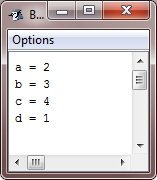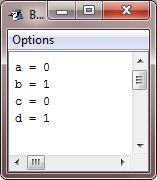# Java Increment and Decrement Operator

The operator (++) and the operator (--) are Java's increment and decrement operators. The increment (++) and decrement operator (--) are simply used to increase and decrease the value by one.

The increment operator adds one to its operand, while the decrement operator subtracts one from its operand. Consider the following statement:

`x = x + 1;`

can be rewritten in this manner by using the increment operator, ++.

`x++;`

Similarly, the following statement:

`x = x - 1;`

can also be rewritten in this manner by using the decrement operator, --.

`x--;`

These operators are special because they can appear both in postfix and prefix form. In postfix form, they come after the operand. In prefix form, they come before the operand.

## Prefix increment operator in Java

The prefix increment operator, of course, increases the value by one, but it does so before it is used. In Java, the prefix increment operator has the following general form:

`++variable;`

For example:

Java Code
```public class PrefixIncrementExample {
public static void main(String[] args) {
int x = 5;
int y = ++x;

System.out.println("x = " + x);
System.out.println("y = " + y);
}
}```
Output
```x = 6
y = 6```

## Postfix increment operator in Java

The postfix increment operator, of course, increases the value by one, but it does so after it is used. In Java, the postfix increment operator has the following general form:

`variable++;`

For example:

Java Code
```public class PrefixIncrementExample {
public static void main(String[] args) {
int x = 5;
int y = x++;

System.out.println("x = " + x);
System.out.println("y = " + y);
}
}```
Output
```x = 6
y = 5```

## Prefix decrement operator in Java

The prefix decrement operator, of course, decreases the value by one, but it does so before it is used. In Java, the prefix decrement operator has the following general form:

`--variable;`

For example:

Java Code
```public class PrefixIncrementExample {
public static void main(String[] args) {
int x = 5;
int y = --x;

System.out.println("x = " + x);
System.out.println("y = " + y);
}
}```
Output
```x = 4
y = 4```

## Postfix decrement operator in Java

The postfix decrement operator, of course, decreases the value by one, but it does so after it is used. In Java, the postfix decrement operator has the following general form:

`variable--;`

For example:

Java Code
```public class PrefixIncrementExample {
public static void main(String[] args) {
int x = 5;
int y = x--;

System.out.println("x = " + x);
System.out.println("y = " + y);
}
}```
Output
```x = 4
y = 5```

## Java Increment and Decrement Operators Example

This Java program demonstrates the increment operator.

```public class JavaProgram {
public static void main(String args[])  {
int a = 1, b = 2, c = ++b, d = a++;
c++;
System.out.println("a = " + a);
System.out.println("b = " + b);
System.out.println("c = " + c);
System.out.println("d = " + d);
}
}```

When the above Java program is compiled and executed, it will produce the following output:Now, this Java program demonstrates the decrement operator:

```public class JavaProgram {
public static void main(String args[])  {
int a = 1, b = 2, c = --b, d = a--;
c--;
System.out.println("a = " + a);
System.out.println("b = " + b);
System.out.println("c = " + c);
System.out.println("d = " + d);
}
}```

When the above Java program is compiled and executed, it will produce the following output:Java Online Test

« Previous Tutorial Next Tutorial »